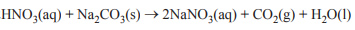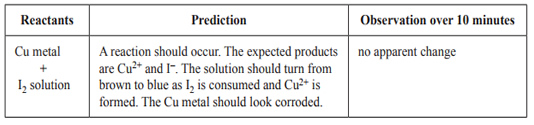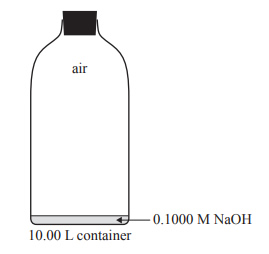General (2016 VCE) 1)Hydrogen peroxide solutions are commercially available and have a range of uses. The active ingredient, hydrogen peroxide, H2O2, undergoes decomposition in the presence of a suitable catalyst according to the reaction 2H2O2(l) → 2H2O(l) + O2(g) In this reaction, oxygen A. only undergoes oxidation. B. only undergoes reduction. C. undergoes both oxidation and reduction. D. undergoes neither oxidation nor reduction. Solution2) Dilute nitric acid reacts with anhydrous sodium carbonate to produce carbon dioxide gas.In an experiment, 0.142 mol anhydrous Na2CO3 powder was added to excess HNO3 in solution, in a 2.00 L reinforced, sealed, metal vessel. Pressure and temperature sensors were used to monitor the reaction. The vessel was initially at 101.3 kPa and 22.0 °C. When the reaction was complete, the final temperature was 24.1 °C. What is the additional pressure, in kPa, inside the vessel due to the carbon dioxide gas after the completion of the reaction? (Assume that the volume of the solution in the vessel is negligible.) A. 349 B. 175 C. 28.3 D. 14.2 Solution3) A class of Chemistry students investigated the reaction of copper metal and iodine solution. After making predictions about the reaction, they placed a copper strip into an iodine solution and compared their predictions with their observations. A number of groups recorded the following.The predicted results were not observed. The class was asked to suggest some hypotheses to explain the unexpected result. Which one of the following hypotheses could not explain the unexpected result? A. The reaction rate might have been too slow for the time allowed. B. An equilibrium was established and [Cu2+] was too low to be visible. C. A bromine solution was accidentally used in place of the iodine solution. D. The surface of the copper metal was greasy. Solution4) Standard solutions of sodium hydroxide, NaOH, must be kept in airtight containers. This is because NaOH is a strong base and absorbs acidic oxides, such as carbon dioxide, CO2, from the air and reacts with them. As a result, the concentration of NaOH is changed to an unknown extent.a. CO2 in the air reacts with water to form carbonic acid, H2CO3. This can react with NaOH to form sodium carbonate, Na2CO3. i. Write a balanced overall equation for the reaction between CO2 gas and water to form H2CO3. Soluiton ii. Write a balanced equation for the complete reaction between H2CO3 and NaOH to form Na2CO3. Solution b. A 10.00 L container is completely filled with a freshly made 0.1000 M NaOH solution. During a Chemistry class, 9.90 L of the solution is used and air enters the empty space above the remaining solution before the container is completely sealed off from the outside air. The container is then opened. Air enters the container at 101.3 kPa and 21.5 °C. Assume that the concentration of CO2 in the air is 0.0400 %(v/v). Solutionc) Calculate the amount of NaOH, in mol, that would be present in the solution that remains in the container. Assume that the NaOH did not react with the CO2 in the air that entered when the container was opened. Solution d) The container is then shaken thoroughly, ensuring that all the CO2 in the air is absorbed into the solution. Calculate the resulting concentration of NaOH in the solution in the container. SolutionExaminers comment for question 4) above.